# Tomography

Reconstruction from projections, i.e. the recovery of a function from its line or (hyper)plane integrals. Important issues are existence, uniqueness and stability of inversion procedures, as well as the development of efficient numerical algorithms. In tomography a fundamental role is played by the Radon transform on Euclidean space. Let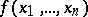be a continuous function of the real variables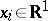that is decreasing sufficiently fast at infinity,;. Letbe a unit vector in,a real number and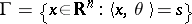a hyperplane, where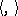denotes the Euclidean inner product. The integral ofover the hyperplane,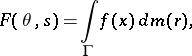(a1)

where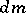is the Euclidean measure on, is called the Radon transform of the function. The Radon operatormaps the space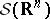of rapidly-decreasing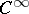-functions onto the space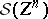of rapidly-decreasing-functions on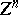, where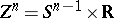is the unit cylinder inandthe unit sphere in. The integral, withandfixed, is called a projection. The Radon transform is connected to the Fourier transform through the central slice theorem: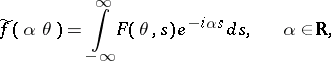(a2)

where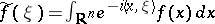denotes the-dimensional Fourier transform of. To the Radon operator is associated a dual or backprojection operator, by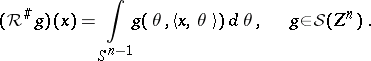(a3)

Whereas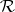integrates over all points in a hyperplane,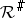integrates over all hyperplanes through a point. Thus,form a dual pair in the sense of integral geometry (see [a1], [a8], [a9]). Related transforms are the-plane transform, which integrates a function over-dimensional subspaces (the case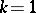is called the-ray transform; the case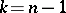is identical to the Radon transform), and the divergent beam transform, which integrates over half-lines.

## Inversion.

The inversion formula of the Radon transform takes two different forms, depending on whether the dimension is even or odd [a12], [a17]: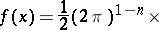Heredenotes the-st derivative ofwith respect to its last (scalar) argument. So, forodd only local information in a neighbourhood of the pointis needed, whereas for eventhe integrals along all hyperplanes meeting the support of the function are required. In his 1917 paper, J. Radon [a17] also discussed the problem of determining a function on the hyperbolic plane from its integrals over all geodesics. Previously, a similar method had been used by P. Funk [a5] for the elliptic case of reconstructing an even function on the sphere from the integrals along the great circles. A unified form of the inversion formula for odd and evenis given by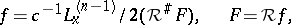(a4)

where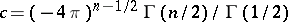(withthe gamma-function and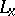the Laplace operator (acting on)), see [a8]. Similar expressions for the dual transform exist. Inversion formulas for the divergent beam and-ray transforms are analogous to those for. Other inversion formulas for the Radon transform are obtained by analytic series expansion methods, see [a3].

From the inversion formulas it follows thatis uniquely determined by. If the transform is only known on a subset of the domain, this may no longer be true. Some of these so-called incomplete data problems are as follows (cf. Potential theory, inverse problems in). i) The exterior problem, i.e. recovering a function in the exterior of some ball from integrals over lines or planes outside that ball. This problem is uniquely solvable, ifis decaying fast enough at infinity. ii) The interior problem, i.e. determininginside some ball from integrals inside that ball. A unique solution exists for odd dimension only. iii) The limited angle problem, whereis given only forin a subset of a half-sphere. Uniqueness holds as long as the set of directions is such that no non-trivial homogeneous polynomial vanishes on it. iv) Problems with a finite number of directions. The solution is non-unique to a very large extent. In fact, for any finite set of directions and any function, witha compact set in, and any compact setin the interior of, one can find a function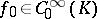which coincides withonand for which all the line integrals along the given directions are identically zero, see [a20]. Such (highly oscillatory) functions, sometimes called "ghostghosts" , form the null-space of the transform for finitely many projections. To resolve this indeterminacy one has to put restrictions on the variation of the function. On the other hand, by increasing the number of projections, arbitrarily good approximations to the unknown function can be found.

## Reconstruction algorithms.

The identity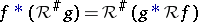, wheredenotes convolution (cf. Convolution of functions), is the basis of filtered backprojection. One choosesin such a way that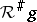is a low-pass filter approaching a delta-distribution, so thatis recovered by a convolution followed by backprojection (both operations properly discretized). Variants exist where the backprojection is performed before the filtering procedure. The central slice theorem is the basis of Fourier reconstruction. Here the projectionsare Fourier transformed with respect to the last variable, which is allowed by the inverse-dimensional Fourier transform. To make use of the fast Fourier transform (cf. Fourier transform, discrete), one first has to interpolate from a polar grid to a Cartesian grid. Analytic series expansion methods are based upon discretization of the inversion formulas for the individual expansion coefficients of the unknown function. Algebraic reconstruction techniques use iterative methods from numerical linear algebra (cf. Iteration methods) applied to the system of linear equations resulting from a full discretization of Radon's integral equation.

Problems in computerized tomography are ill-posed (cf. Ill-posed problems). There may be no solution if the data are not in the range of the transform, or the solution may be non-unique, problems which can be overcome by using the concept of generalized inverses. Moreover, the operator which maps the transform to the solution may not be continuous. In that case one uses regularization procedures, such as Tikhonov–Phillips regularization, iterative methods with appropriate stopping criteria or truncated singular-value decompositions [a4], [a15]. Limited data problems in particular are severely ill-posed. In this context one also may apply various statistical estimation procedures with or without the use of a-priori information, often resulting in iterative algorithms.

## Generalizations.

Generalized Radon transforms can be defined, where the integration is over subspaces or, more generally, over manifolds [a6], [a8], [a12]. A related problem for such spaces is the orbital integral problem, i.e. recovering a function from its integrals over (generalized) spheres. In acoustic or diffraction tomography, which is a special inverse scattering problem,can be recovered from data on semi-circles through the origin (see [a14], [a18], [a21]). Another variant is the attenuated Radon transform, which differs from the standard Radon transform by the presence of an exponential weighting factor under the integral sign. For the use of the Radon transform in the area of partial differential equations, see [a8], [a13]. One more generalization of Radon's problem is the reconstruction of a measure from its projections, i.e. from lower-dimensional measures induced by some measurable mapping. For the Radon transform in the complex domain, see [a6]. Finite Radon transforms have also been studied.

## Applications.

Tomography is a widely used method to reconstruct cross-sections of the interior structure of an object without having to cut or damage the object. In this context one usually speaks of computerized (computed, computer assisted) tomography, since for actually performing the reconstructions in practice one needs to use a digital computer. Through the interaction of a physical agens or "probe" — varying from-rays, gamma rays, visible light, electrons, or neutrons to ultrasound waves or nuclear magnetic resonance signals — with the object, one obtains line or plane integrals of the internal distribution to be reconstructed. One of the most prominent examples of computerized tomography occurs in diagnostic medicine, where the method is used to produce images of the interior of human organs. In industry the method is used for non-destructive testing. Other applications arise in radio astronomy, radar theory, electron microscopy, aerodynamics, geophysics, and oceanography, see [a3], [a10], [a14] for references. Finite Radon transforms are used in various areas such as the theory of error-correcting codes and lattice theory.